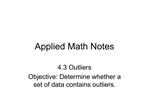# Applied Math Notes - PowerPoint PPT PresentationDownload PresentationApplied Math Notes

Applied Math Notes
Download Presentation## Applied Math Notes

- - - - - - - - - - - - - - - - - - - - - - - - - - - E N D - - - - - - - - - - - - - - - - - - - - - - - - - - -
##### Presentation Transcript

1. Applied Math Notes 4.3 Outliers Objective: Determine whether a set of data contains outliers.

3. Testing for Outliers using Quartiles and IQR If a data value is more than 1.5 interquartile ranges below the lower quartile, then it is a lo-end outlier. Q1 � 1.5IQR is the lo-end cutoff value using quartiles If a data value is more than 1.5 interquartile ranges above the upper quartile, then it is a hi-end outlier. Q3 + 1.5IQR is the hi-end cutoff value using quartiles

4. Example 1: Finding outliers using quartiles Use the following data set to determine whether there are any outliers using quartiles: 16, 25, 24, 19, 33, 25, 34, 46, 37, 33, 42, 40, 37, 34, 49, 73

5. Testing for Outliers using the Mean and Standard Deviation If a data value is more than 3 standard deviations below the mean, then it is a lo-end outlier. � - 3s is the lo-end cutoff value using mean and standard deviation. If a data value is more than 3 standard deviations above the mean, then it is a hi-end outlier. � + 3s is the hi-end cutoff value using mean and standard deviation.

6. Example 2: Finding outliers using mean and standard deviation. Use the mean and standard deviation to identify any outliers from the following data set: 61, 92, 83, 63, 93, 69, 72, 74, 75, 32, 80, 83, 86, 88, 75, 78, 88, 89, 97, 74